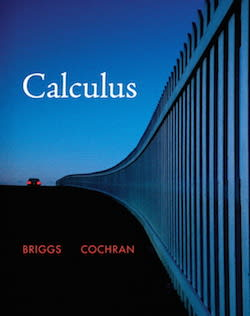Calculus Calculus: Early Transcendentals# Calculus: Early Transcendentals Briggs • 1st Edition • 978-0321336118

Not the textbook you were looking for? Pick another one here.

## Ch.1: Functions (Part 1)

Representation of a Function Word Problems as Functions Types of Functions Asymptotes Rational Functions Computing Domain Domain & Range

## Ch.1: Functions (Part 2)

Evaluating Functions Composition of Functions Transformations of Functions Symmetry Piece-Wise Functions

## Ch.1: Functions (Part 3)

Inverse Functions Exponential Functions Logarithmic Functions

## Ch.2: Limits (Part 1)

Introduction to Limits Limits at Infinity Computing Limits Limits of Trigonometric Functions

## Ch.2: Limits (Part 2)

Infinite Limts Continuity

## Ch.3: Derivatives (Part 1)

Intro to Derivative (Long Way) Power Rule Product Rule Quotient Rule Chain Rule

## Ch.3: Derivatives (Part 2)

Derivatives Trigonometric Functions Derivatives of Inverse Trigonometric Functions Derivatives of Exponential Functions Derivatives Of Logarithmic Functions

## Ch.3: Derivatives (Part 3)

Implicit Differentiation Logarithmic Differentiation Related Rates

## Ch.4: Applications of the Derivative (Part 1)

Extremas Concavity Curve Sketching

## Ch.4: Applications of the Derivative (Part 2)

Optimization Linear Approximations & Differentials Newton's Method Theorems (MVT) L'Hopital's Rule

## Ch.5: Integration

Area The Definite Integral Indefinite Integrals The Substitution Rule